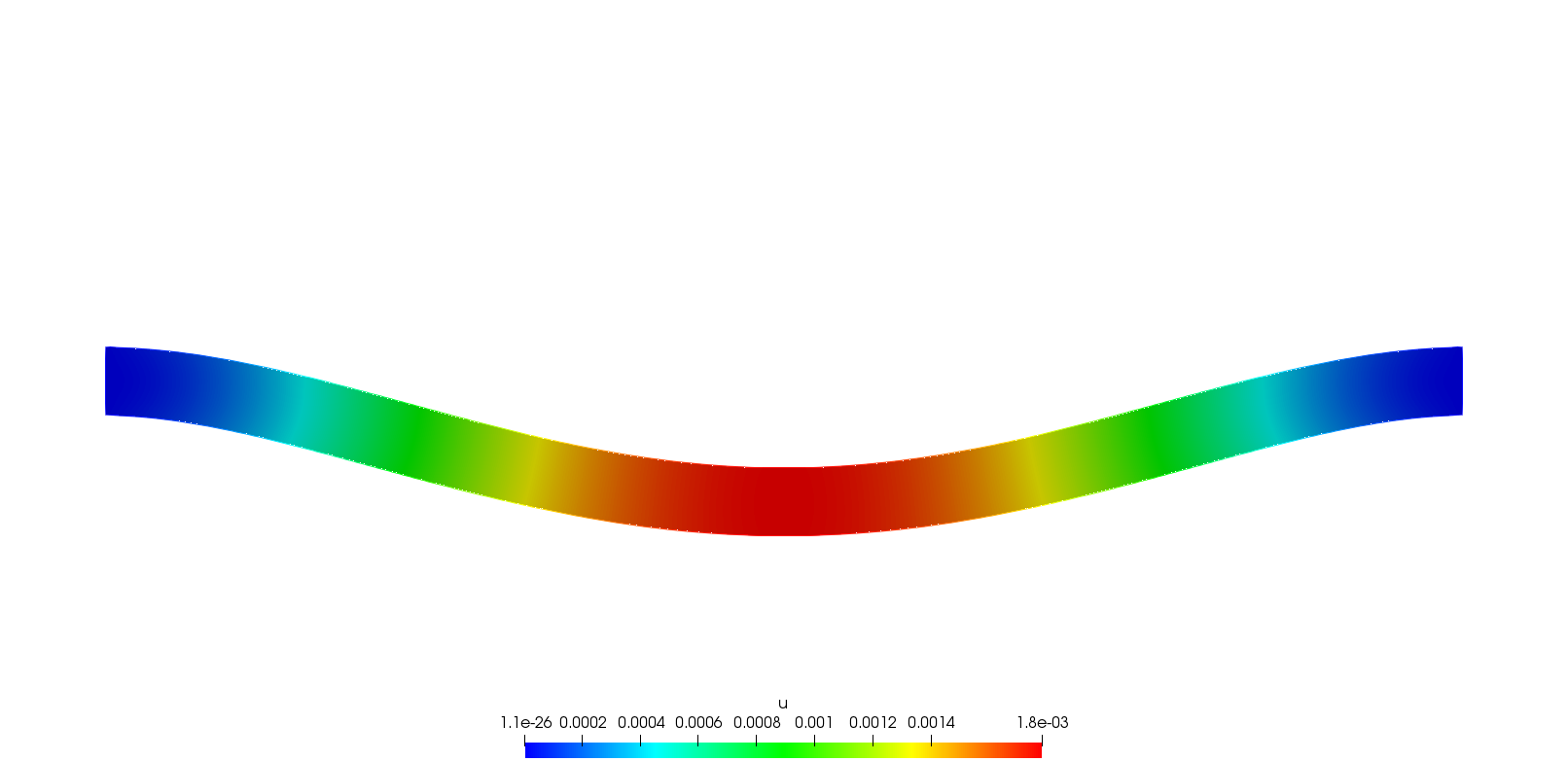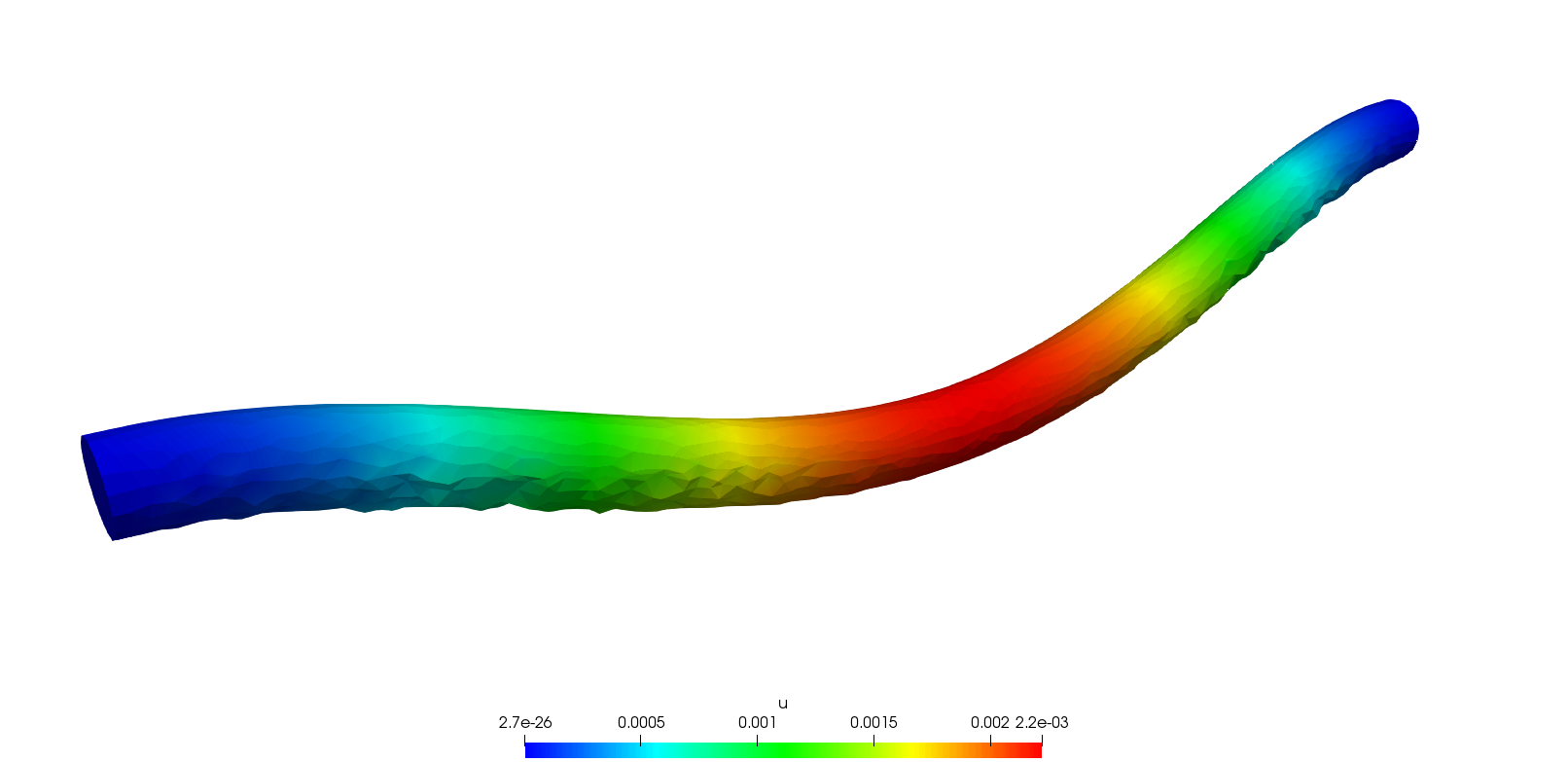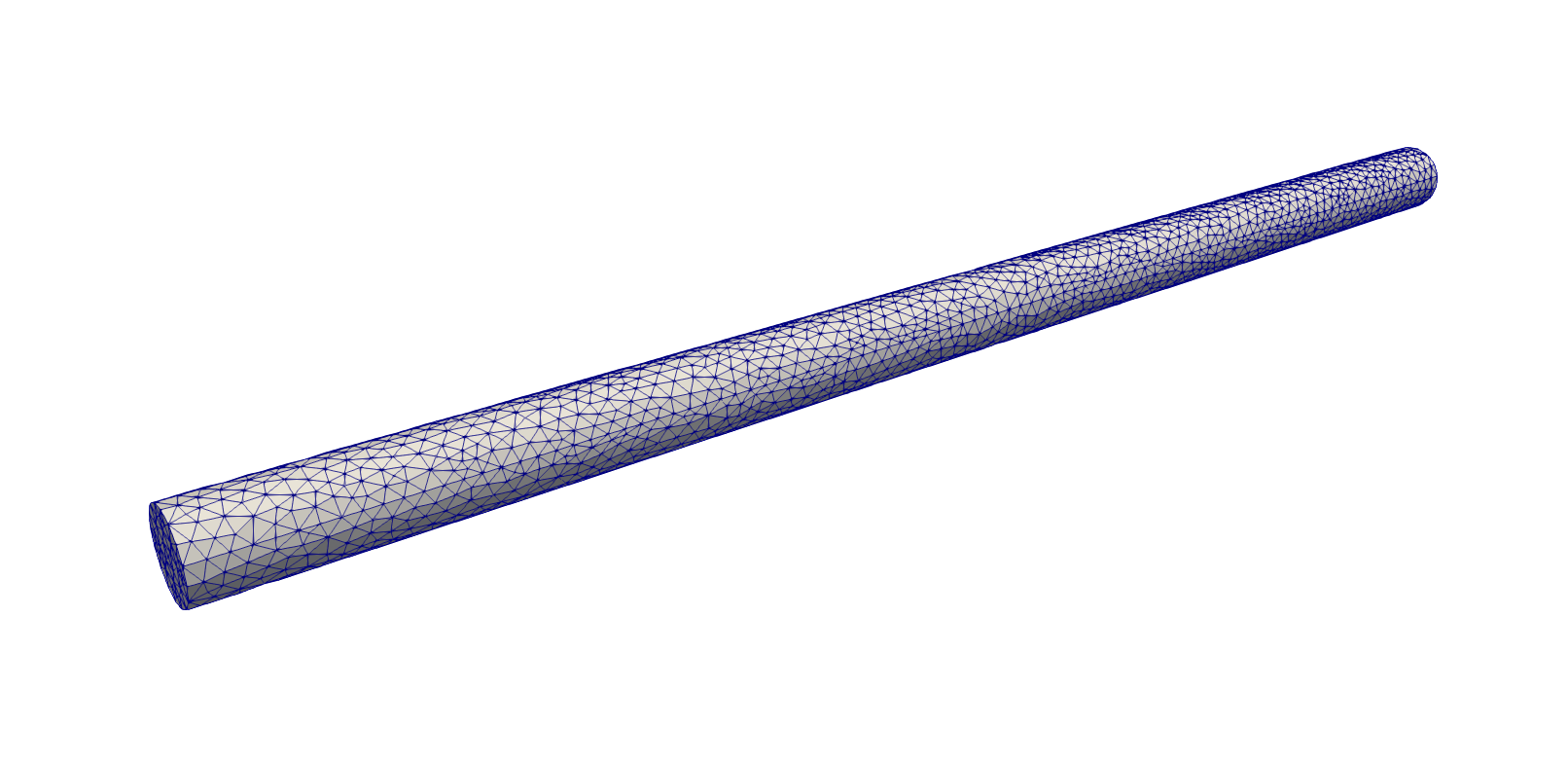Elasticity

Algorithms for solving the 2D and 3D static linear elasticity equations

Problem

Solve:

$\displaystyle{ -\nabla\cdot\sigma = \mathbf{f} }$

With:

$\displaystyle{ \sigma_{ij}(\mathbf{u}) = \lambda\delta_{ij}\nabla\cdot\mathbf{u} + 2\mu\varepsilon_{ij}(\mathbf{u}) }$

and

$\displaystyle{ \mu = \frac{E}{2(1+\nu)} ;\ \lambda = \frac{E\nu}{(1+\nu)(1-2\nu)} }$

Variational form

Let $\Omega\in\mathbb{R}^n$, $2\leq n\leq3$. Let $\mathbf{u}, \mathbf{v}\in \left(H^1(\Omega)\right)^n$. The variational form reads as follows:

$\displaystyle{ \int_{\Omega}{\lambda\nabla\cdot\mathbf{u}\nabla\cdot\mathbf{v} + 2\mu\varepsilon(\mathbf{u})}:\varepsilon(\mathbf{v}) - \int_{\Omega}{\mathbf{f}\cdot\mathbf{v}} = 0 }$

Algorithms

2D

Elasticity equation on a beam.

Result warped by a factor 10003D

Elasticity equation on a beam.

Result warped by a factor 1000Optional

Gmsh script:

MeshTODO

Authors

Author: Simon Garnotel